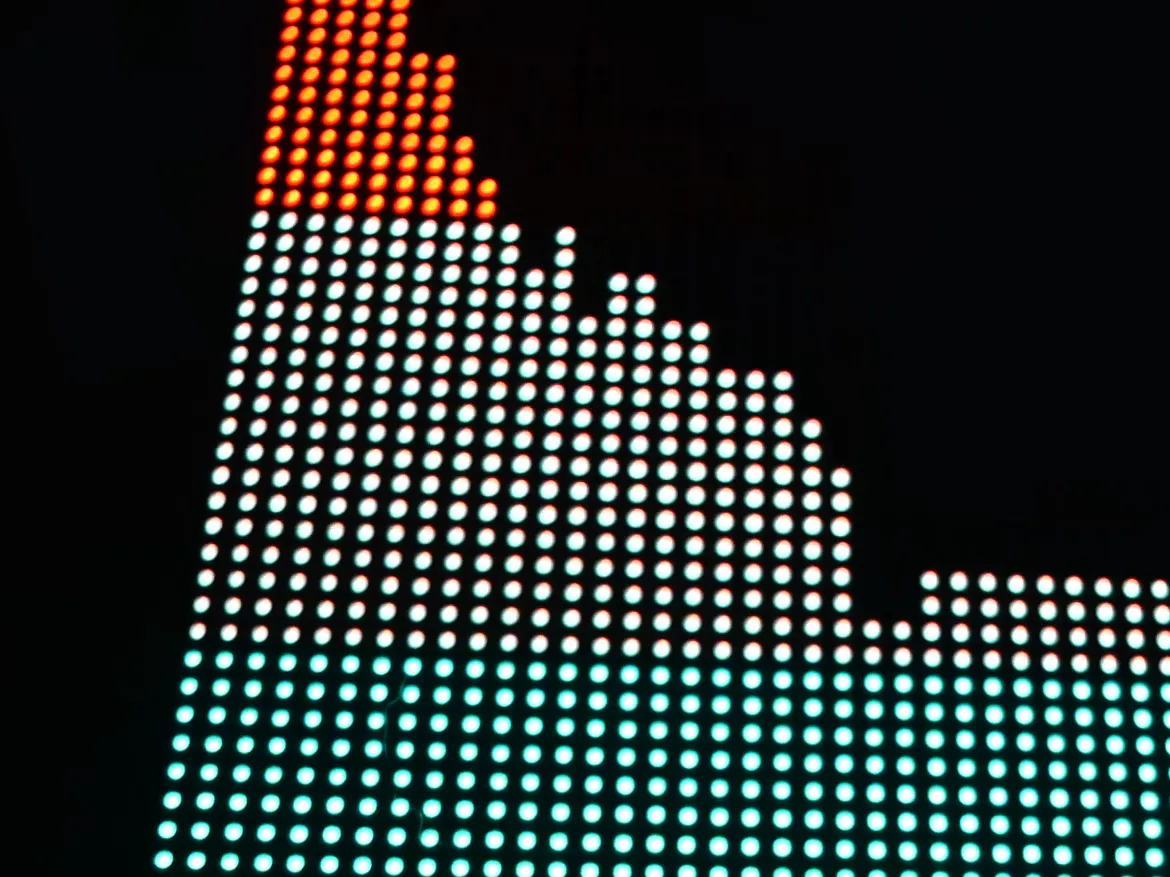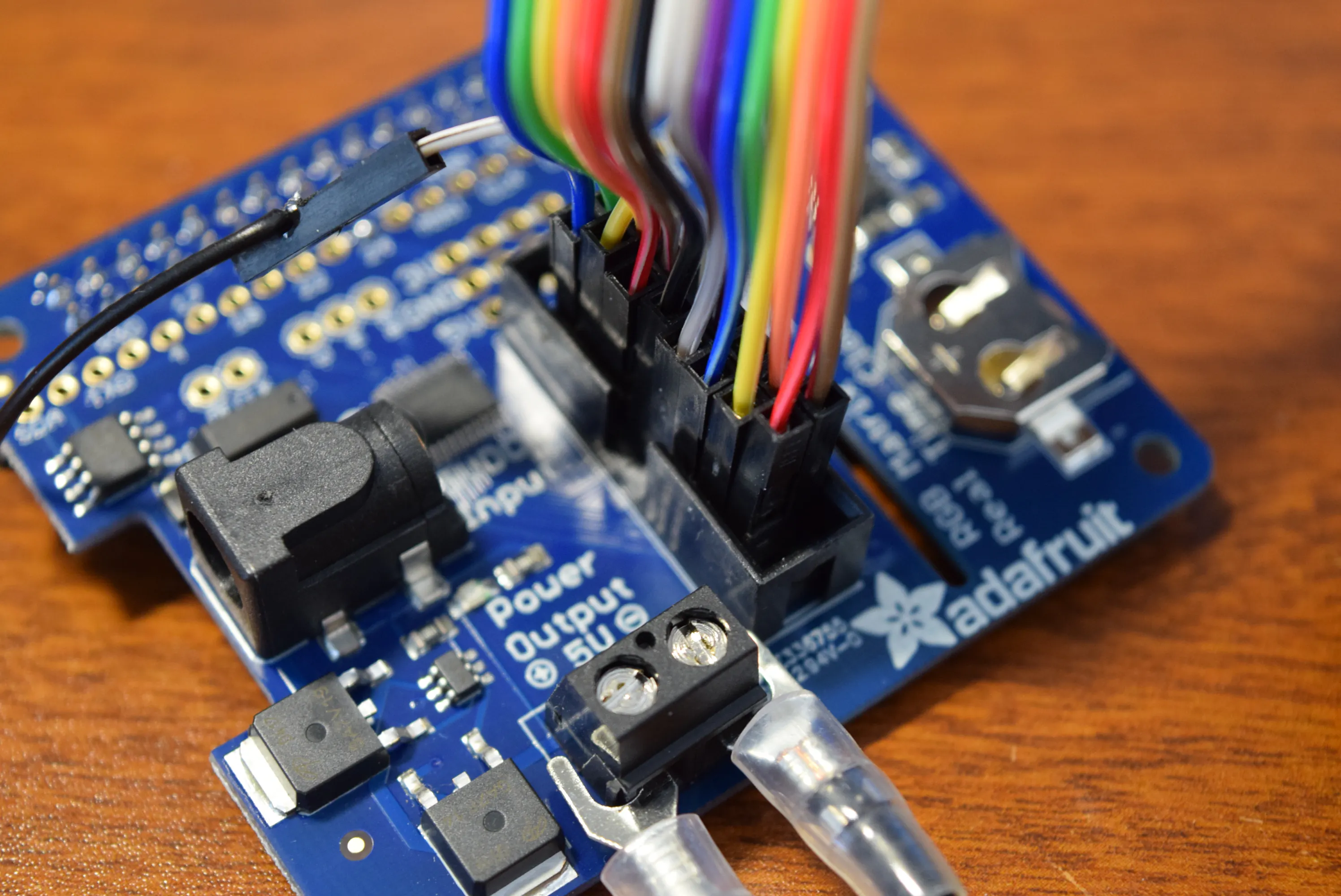# Raspberry Pi Audio Spectrum Display

Use the DFRobot 64x64 RGB matrix panel with a Raspberry Pi 3 B+ to bring a dance party with you wherever you go!

IntermediateFull instructions provided2 hours11,487## Things used in this project

### Hardware components

 DFRobot 64 x 64 RGB LED Matrix
×1
 DFRobot Raspberry Pi 3 B+
×1
 Adafruit RGB Matrix HAT
×1
 DFRobot ESP32 FireBeetle
×1

### Software apps and online services

 Raspbian
 Python 2.7
 FileZIlla

### Hand tools and fabrication machinesSoldering iron (generic)

## Schematics

### Board## Code

### Pi Code

Python
```import alsaaudio as aa
import wave
from struct import unpack
import numpy as np
from rgbmatrix import RGBMatrix, RGBMatrixOptions, graphics

wavfile = wave.open('test.wav')
sample_rate = wavfile.getframerate()
no_channels = wavfile.getnchannels()

options = RGBMatrixOptions()
options.rows = 64
options.cols = 64
options.chain_length = 1
options.parallel = 1
Dmatrix = RGBMatrix(options=options)
Dmatrix.Clear()

chunk = 4096

matrix =  * 64

weighting = *64
weighting = 2
power = []

for i in range(63):
if i%2==0:
weighting[i+1] = 2**(2+i)
else:
weighting[i+1] = 2**(1+i)

output = aa.PCM(aa.PCM_PLAYBACK,aa.PCM_NORMAL)
output.setchannels(2)
output.setrate(sample_rate)
output.setformat(aa.PCM_FORMAT_S16_LE)
output.setperiodsize(chunk)

def piff(val):
return int(2*chunk*val/sample_rate)

def calculate_levels(data, chunk, sample_rate):
data = unpack("%dh"%(len(data)/2),data)
data = np.array(data,dtype='h')
fourier = np.fft.rfft(data)
fourier = np.delete(fourier,len(fourier)-1)
power = np.log10(np.abs(fourier))**2
power = np.reshape(power,(64,chunk/64))
matrix = np.int_(np.average(power,axis=1))
return matrix

while data != '':
output.write(data)
matrix = calculate_levels(data,chunk,sample_rate)
#print(matrix)
Dmatrix.Clear()
for y in range(0,64):
for x in range(matrix[y]):
x *=2
if x < 32:
Dmatrix.SetPixel(y,x,0,200,0)
Dmatrix.SetPixel(y,x-1,0,200,0)
elif x < 50:
Dmatrix.SetPixel(y,x,150,150,0)
Dmatrix.SetPixel(y,x-1,150,150,0)
else:
Dmatrix.SetPixel(y,x,200,0,0)
Dmatrix.SetPixel(y,x-1,200,0,0)